Python建模：预测周期性时间序列的正确姿势

数据探索

print('size: ',data.shape)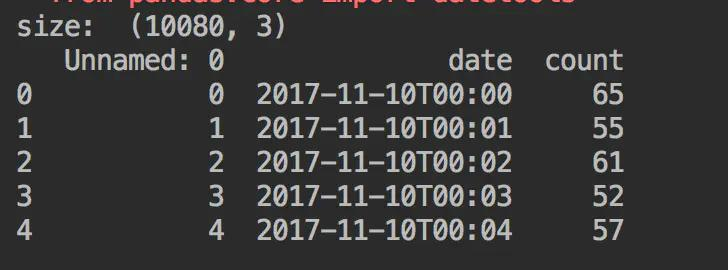date：时间，单位分钟

count：该分钟该api被访问的次数

def draw_ts(timeseries):
timeseries.plot()
plt.show()
​
data = data.set_index('date')
data.index = pd.to_datetime(data.index)
ts = data['count']
draw_ts(ts)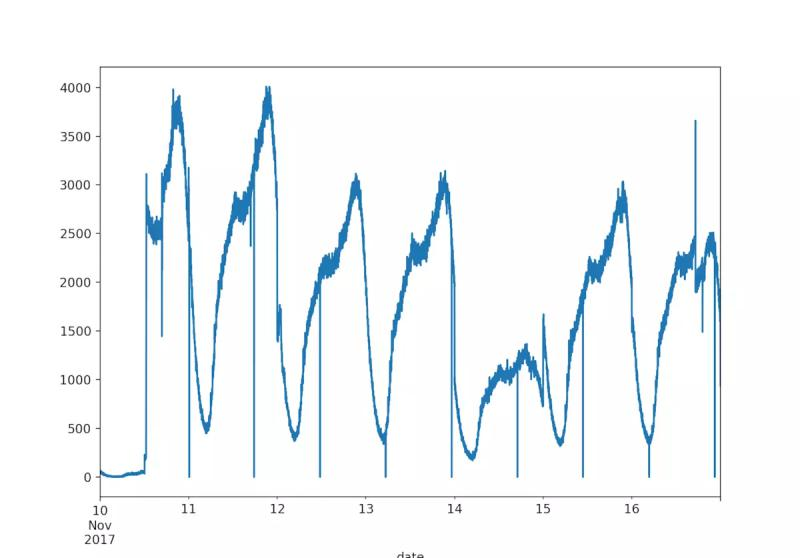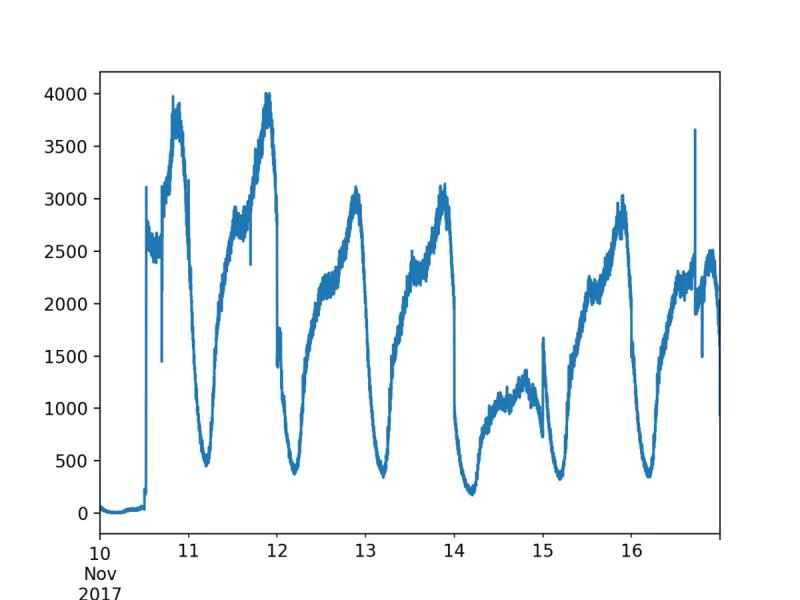1、这是一个周期性的时间序列，数值有规律的以天为周期上下波动，图中这个api，在每天下午和晚上访问较为活跃，在早上和凌晨较为稀少。在建模之前需要做分解。

2、我的第二个坑：数据本身并不平滑，骤突骤降较多，而这样是不利于预测的，毕竟模型需要学习好正常的序列才能对未知数据给出客观判断，否则会出现频繁的误报，令气氛变得十分尴尬( ´Д｀)，所以必须进行平滑处理。

3、这只是一个api的序列图，而不同的api的形态差距是很大的，毕竟承担的功能不同，如何使模型适应不同形态的api也是需要考虑的问题。

预处理

1、划分训练测试集

class ModelDecomp(object):
def __init__(self, file, test_size=1440):
self.test_size = test_size
self.train_size = len(self.ts) - self.test_size
self.train = self.ts[:len(self.ts)-test_size]
self.test = self.ts[-test_size:]

2、对训练数据进行平滑处理

def _diff_smooth(self, ts):
dif = ts.diff().dropna() # 差分序列
td = dif.describe() # 描述性统计得到：min，25%，50%，75%，max值
high = td['75%'] + 1.5 * (td['75%'] - td['25%']) # 定义高点阈值，1.5倍四分位距之外
low = td['25%'] - 1.5 * (td['75%'] - td['25%']) # 定义低点阈值，同上
​
# 变化幅度超过阈值的点的索引
forbid_index = dif[(dif > high) | (dif < low)].index
i = 0
while i < len(forbid_index) - 1:
n = 1 # 发现连续多少个点变化幅度过大，大部分只有单个点
start = forbid_index[i] # 异常点的起始索引
while forbid_index[i+n] == start + timedelta(minutes=n):
n += 1
i += n - 1
​
end = forbid_index[i] # 异常点的结束索引
# 用前后值的中间值均匀填充
value = np.linspace(ts[start - timedelta(minutes=1)], ts[end + timedelta(minutes=1)], n)
ts[start: end] = value
i += 1
​
self.train = self._diff_smooth(self.train)
draw_ts(self.train)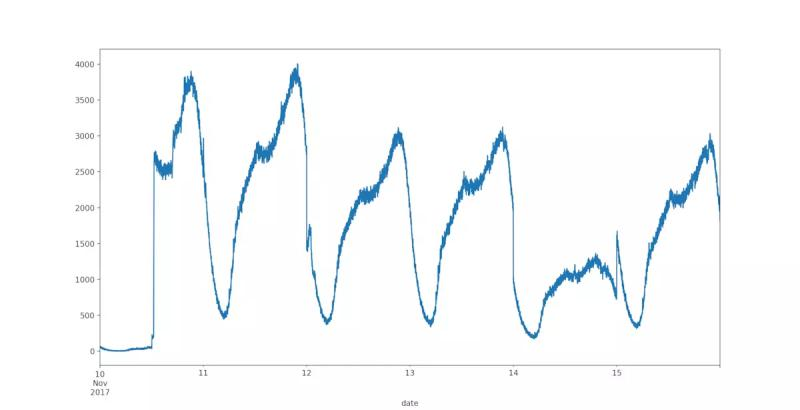3、将训练数据进行周期性分解

from statsmodels.tsa.seasonal import seasonal_decompose
​
decomposition = seasonal_decompose(self.ts, freq=freq, two_sided=False)
# self.ts:时间序列，series类型;
# freq:周期，这里为1440分钟，即一天;
# two_sided:观察下图2、4行图，左边空了一段，如果设为True，则会出现左右两边都空出来的情况，False保证序列在最后的时间也有数据，方便预测。
​
self.trend = decomposition.trend
self.seasonal = decomposition.seasonal
self.residual = decomposition.resid
decomposition.plot()
plt.show()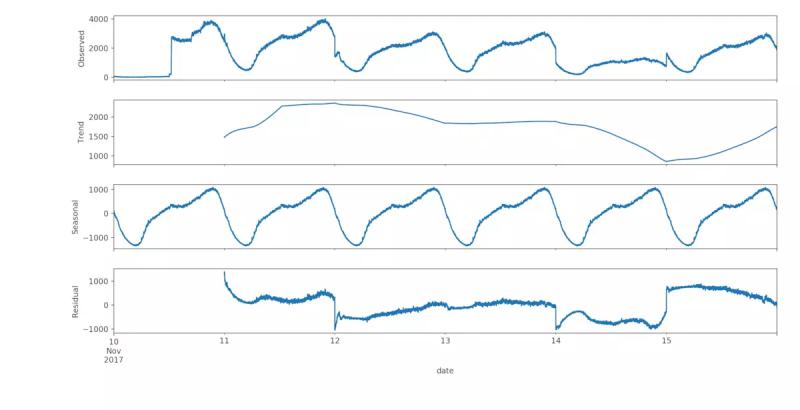模型

1、训练

def trend_model(self, order):
self.trend.dropna(inplace=True)
train = self.trend[:len(self.trend)-self.test_size]
#arima的训练参数order =（p,d,q），具体意义查看官方文档，调参过程略。
self.trend_model = ARIMA(train, order).fit(disp=-1, method='css')

2、预测

d = self.residual.describe()
delta = d['75%'] - d['25%']
self.low_error, self.high_error = (d['25%'] - 1 * delta, d['75%'] + 1 * delta)

def predict_new(self):
'''
预测新数据
'''
#续接train，生成长度为n的时间索引，赋给预测序列
n = self.test_size
self.pred_time_index= pd.date_range(start=self.train.index[-1], periods=n+1, freq='1min')[1:]
self.trend_pred= self.trend_model.forecast(n)
​
​
'''
为预测出的趋势数据添加周期数据和残差数据
'''
self.train_season = self.seasonal[:self.train_size]
values = []
low_conf_values = []
high_conf_values = []
​
for i, t in enumerate(self.pred_time_index):
trend_part = self.trend_pred[i]
​
# 相同时间点的周期数据均值
season_part = self.train_season[
self.train_season.index.time == t.time()
].mean()
​
# 趋势 + 周期 + 误差界限
predict = trend_part + season_part
low_bound = trend_part + season_part + self.low_error
high_bound = trend_part + season_part + self.high_error
​
values.append(predict)
low_conf_values.append(low_bound)
high_conf_values.append(high_bound)
​
# 得到预测值，误差上界和下界
self.final_pred = pd.Series(values, index=self.pred_time_index, name='predict')
self.low_conf = pd.Series(low_conf_values, index=self.pred_time_index, name='low_conf')
self.high_conf = pd.Series(high_conf_values, index=self.pred_time_index, name='high_conf')

3、评估：

md = ModelDecomp(file=filename, test_size=1440)
md.decomp(freq=1440)
md.trend_model(order=(1, 1, 3)) # arima模型的参数order
md.predict_new()
pred = md.final_pred
test = md.test
​
plt.subplot(211)
plt.plot(md.ts) # 平滑过的训练数据加未做处理的测试数据
plt.title(filename.split('.'))
​
plt.subplot(212)
pred.plot(color='blue', label='Predict') # 预测值
test.plot(color='red', label='Original') # 真实值
md.low_conf.plot(color='grey', label='low') # 低置信区间
md.high_conf.plot(color='grey', label='high') # 高置信区间
​
plt.legend(loc='best')
plt.title('RMSE: %.4f' % np.sqrt(sum((pred.values - test.values) ** 2) / test.size))
plt.tight_layout()
plt.show()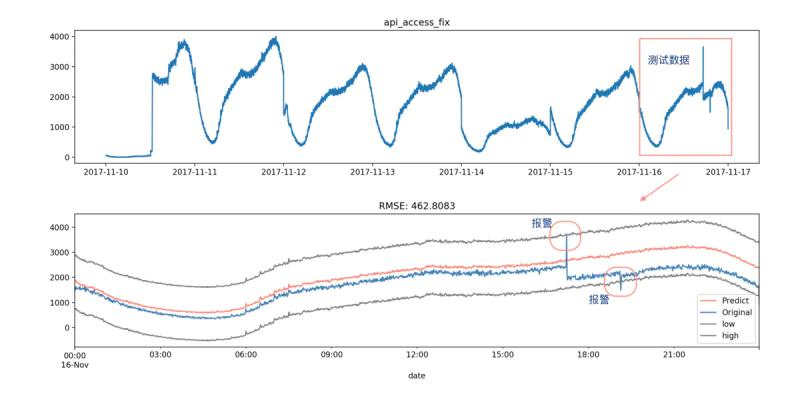结语

https://www.jianshu.com/p/09e5218f58b4

https://shimo.im/docs/JWCghr8prjCVCxxK/ 《Python学习资料》02-12

03-172356
05-023665
02-012282
05-042万+
03-061万+
09-03988
05-115682
12-132万+
01-28530
09-305706
07-17192
10-10533
08-171万+
11-1046
06-112万+
10-27704
12-05339点击重新获取扫码支付余额充值